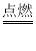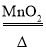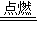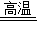↑ 收起筛选 ↑

A. 2H2+O22H2O

B. Zn+H2SO4＝ZnSO4+H2↑

C. 2KClO32KCl+3O2↑

D. KOH+HCl＝KCl+H2O

• 下列反应属于分解反应的是（　　）

A. 2H2+O22H2O

B. Zn+H2SO4＝ZnSO4+H2↑

C. 2KClO32KCl+3O2↑

D. KOH+HCl＝KCl+H2O

九年级化学单选题简单题查看答案及解析

• 下列反应属于分解反应的是（　　）

A. 2H2+O22H2O

B. Zn+H2SO4＝ZnSO4+H2↑

C. 2KClO32KCl+3O2↑

D. KOH+HCl＝KCl+H2O

九年级化学单选题简单题查看答案及解析

• 下列化学反应中，属于分解反应的是（ ）
A．2H2+O22H2O
B．CaCO3CaO+CO2
C．Zn+H2SO4═ZnSO4+H2
D．KOH+HCl═KCl+H2O

九年级化学选择题中等难度题查看答案及解析

• 下列化学反应中，属于分解反应的是（ ）
A．2H2+O22H2O
B．CaCO3CaO+CO2
C．Zn+H2SO4═ZnSO4+H2
D．KOH+HCl═KCl+H2O

九年级化学选择题中等难度题查看答案及解析

• 下列化学反应中，属于分解反应的是（ ）
A．2H2+O22H2O
B．CaCO3CaO+CO2
C．Zn+H2SO4═ZnSO4+H2
D．KOH+HCl═KCl+H2O

九年级化学选择题中等难度题查看答案及解析

• 下列化学反应中，属于分解反应的是（ ）
A．2H2+O22H2O
B．CaCO3CaO+CO2
C．Zn+H2SO4═ZnSO4+H2
D．KOH+HCl═KCl+H2O

九年级化学选择题中等难度题查看答案及解析

• 下列化学反应中，属于分解反应的是（ ）
A．2H2+O22H2O
B．CaCO3CaO+CO2
C．Zn+H2SO4═ZnSO4+H2
D．KOH+HCl═KCl+H2O

九年级化学选择题中等难度题查看答案及解析

• 下列化学反应中，属于分解反应的是（ ）
A．2H2+O22H2O
B．CaCO3CaO+CO2
C．Zn+H2SO4═ZnSO4+H2
D．KOH+HCl═KCl+H2O

九年级化学选择题中等难度题查看答案及解析

• 下列化学反应中，属于分解反应的是（ ）
A．2H2+O22H2O
B．CaCO3CaO+CO2
C．Zn+H2SO4═ZnSO4+H2
D．KOH+HCl═KCl+H2O

九年级化学选择题中等难度题查看答案及解析

• 下列化学反应中，属于分解反应的是（ ）
A．2H2+O22H2O
B．CaCO3CaO+CO2
C．Zn+H2SO4═ZnSO4+H2
D．KOH+HCl═KCl+H2O

九年级化学选择题中等难度题查看答案及解析